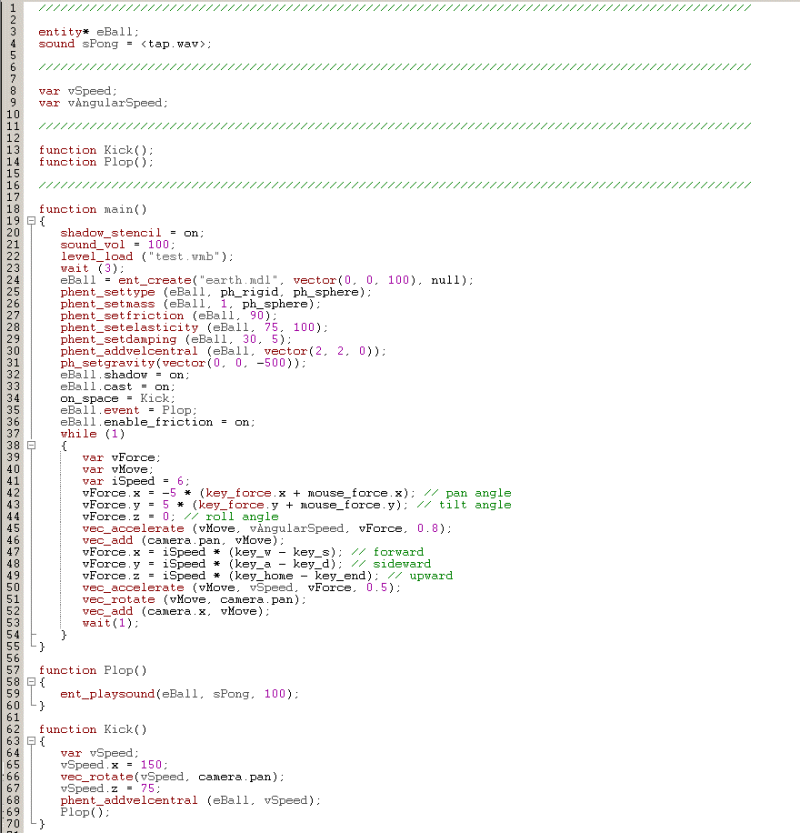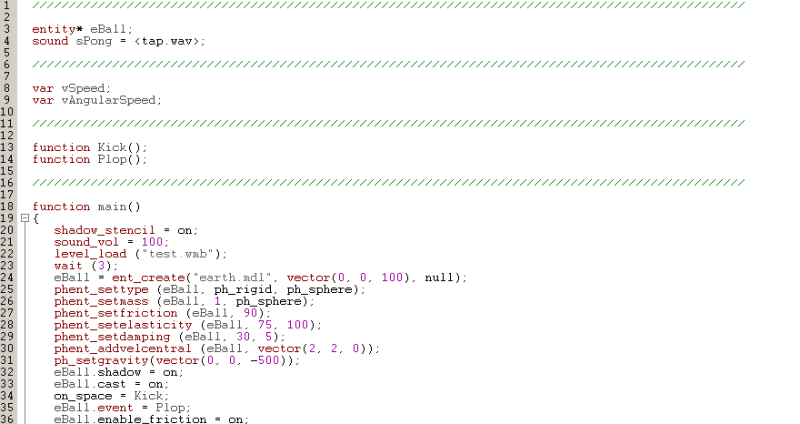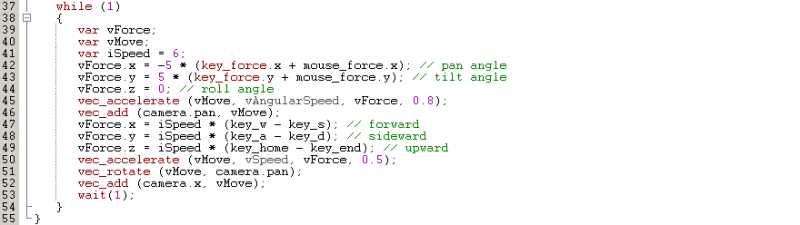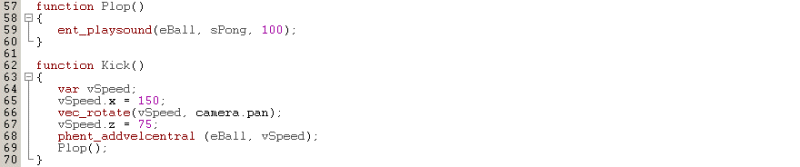Earthball
 If the name of the article sounds familiar, you are right: the code I'm using here is a conversion of the lite-C "earthball" sample that comes with some of the newer versions of the engine. Btw, I have included the earthball.c file in the code archive as well.   Let's run the demo first; open the test.wmp level, and then run it using earthball.wdl; you will see a big room that contains the (earth) ball. Move close to it and then press the "space" key - if you own A6 commercial or A6 pro you will see that the ball jumps as if an invisible force was applied on it. Well, in fact, an invisible force was indeed applied to it! Move the camera where you want it; it can penetrate the walls, so you should be able to apply that invisible force everywhere. And you don't even need to be close to the ball in order to do that!   This snippet sounds like a good start for a basketball game; let's see the entire code at once:It's not that big and it doesn't look complicated at all! Let's break it into smaller pieces now.First of all, we are defining an entity pointer to our ball (eBall), a sound, two global variables and two function prototypes. Function main() holds most of the code inside its greedy brackets: it activates the stencil buffer shadows, it sets the sound volume to 100, loads our level, waits until it is loaded, and then creates the ball entity.   The eBall entity is created using the earth.mdl model file, at a position that's given by x = 0, y = 0, z = 100 and doesn't have any function attached to it because the last function parameter is set to "null". The following lines of code turn our inert ball model into a true physics entity:   - phent_settype (eBall, ph_rigid, ph_sphere) tells our ball to become a physics entity, with a rigid body (water surfaces can be created as well) and with a collision hull that behaves like a sphere. You see, the shape of our model doesn't set its proper physics behavior automatically. If I'd simply replace my earth.mdl sphere with a cube, the brand new cube would continue to rotate and roll as if it were a sphere because phent_settype has told it to behave like one, get it?   - phent_setmass (eBall, 1, ph_sphere) sets the mass of our sphere to 1 kilogram (2.2 lbs).   - phent_setfriction (eBall, 90) sets the friction coefficient to 90. The value can range from 0 (ice-like friction) to 100 (rubber-like friction).   - phent_setelasticity (eBall, 75, 100) sets the bounciness factor for our ball to 75 and the minimum speed to 100. What's with this minimum speed? If the ball has a speed that's lower than 100 when it collides with something, its bounciness will be set to zero - this way you can prevent lots of small, useless bounces from appearing.   - phent_setdamping (eBall, 30, 5) sets the linear damping to 30 and the angular damping to 5. It's a simple and yet effective method to simulate airdrag and friction; without damping, our ball would continue to rotate ad infinitum.   - phent_addvelcentral (eBall, vector(2, 2, 0)) add a small, linear velocity to our ball's center. Without gravity and damping, our ball will be driven by this speed vector. In fact, this line of code moves the ball towards the corner of the level because the speed on x = 2, y = 2 and z = 0 at game start.   - ph_setgravity(vector(0, 0, -500)) sets the global gravity for our ball. Normal values for the gravity vector include x = 0, y = 0 and z set to a negative value, which makes the object fall downwards. For a bit of fun, try to replace -500 with 500 and see what happens.   These are the lines that set our ball properly! The following two lines enable its shadow and suppress the potential self-shadows. We bind function Kick() (more about it a bit later) to the space key, we set function Plop() as the event function for our ball, and then we enable friction-based events for the ball (similar to the collision events for the non-physics entities).   Let's examine the second part of function main() now:This is the code that makes the camera move around and rotate; it sets vForce to the values that are given by the key_force (arrow) keys and / or by the mouse, allowing us to rotate the camera around. Vec_accelerate calculates the proper distance (in quants) for a given speed and acceleration, taking into account a friction factor of 0.8; the result is added to camera.pan, making the actual rotations happen.   We are using the "W", "S", "A", "D" keys to move around in the level and the "Home" and "End" keys to move up and down; change iSpeed if you want to edit the movement speed. The camera movement code is similar with the camera rotation code; the only notable difference appears because of the vec_rotate instruction, which will move the camera in  the direction that's faced by the player (camera.pan).Plop() is ball's event function; it simply plays a sound with a volume of 100 when our ball collides with something. Function Kick() is run each and every time when the player presses the "space" key: it creates a vector that has a length of 150 on the x axis, orients it along camera's pan angle, it then sets the third component of the vector to 75 on the z axis and applies it to our ball, making it move forward and jump when the "space" key is pressed. You are right, if you'd want the ball to move more like a frog you'd set vSpeed.x to (let's say) 30 and vSpeed.z to (let's say) 100. The last line of code calls function Plop(), making it create the ball bouncing sound every time when "space" is being pressed.   I hope that you are now encouraged to learn more about the physics engine. Read the manual to see the other functions that are available, and most of all - make experiments!